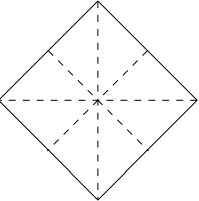# Hypergeometric bootstrapping: implement one, get seven free

Suppose you have software to compute one hypergeometric function. How many more hypergeometric functions can you compute from it?

Hypergeometric functions satisfy a lot of identities, so you can bootstrap one such function into many more. That’s one reason they’re so useful in applications. For this post, I want to focus on just three formulas, the so-called linear transformation formulas that relate one hypergeometric function to another. (There are more linear formulas that relate one hypergeometric function to a combination of two others. I may consider those in a future post, but not today.)

## Linear transformations

The classical hypergeometric function has three parameters. The linear transformations discussed above relate the function with parameters (abc) to those with parameters

• (c – ac – bc)
• (ac – bc)
• (bc – ac)

Here are the linear transformations in detail:## How many more?

The three transformations above are the ones listed in Handbook of Mathematical Functions by Abramowitz and Stegun. How many more transformations can we create by combining them? To answer this, we represent each of the transformations with a matrix. The transformations correspond to multiplying the matrix by the column vector  (abc).The question of how many transformations we can come up with can be recast as asking what is the order of the group generated by AB, and C. (I’m jumping ahead a little by presuming these matrices generate a group. Conceivably the don’t have inverses, but in fact they do. The inverses of AB, and C are AB, and AC respectively.)

A little experimentation reveals that there are eight transformations: I, ABCDABEACFBC, and GCB. So in addition to the identity I, the do-nothing transformation, we have found four more: DEF, and G. These last four correspond to taking  (abc) to

• (c – abc)
• (ac – bc)
• (bac)
• (c – bc – ac)

This means that if we have software to compute the hypergeometric function with one fixed set of parameters, we can bootstrap that into computing the hypergeometric function with up to seven more sets of parameters. (Some of the seven new combinations could be repetitive if, for example, ab.)

## Identifying the group

We have uncovered a group of order 8, and there are only 5 groups that size. We should be able to find a familiar group isomorphic to our group of transformations.

The five groups of order 8 are Z8 (cyclic group), Z4×Z2 (direct product), E8 (elementary Abelian group), D8 (dihedral group), and the quaternion group. The first three are Abelian, and our group is not, so our group must be isomorphic to either the quaternion group or the dihedral group. The quaternion group has only 1 element of order 2, and the dihedral group has 5 elements of order 2. Our group has five elements of order 2 (ABDFG) and so it must be isomorphic to the dihedral group of order 8, the rotations and reflections of a square. (Some authors call this D8, because the group has eight elements. Others call it D4, because it is the group based on a 4-sided figure.)Related: Applied complex analysis

## One thought on “Hypergeometric bootstrapping: implement one, get seven free”

1. Ah, hypergeometric!

And the hypergeometric distribution (from statistics) has a weighted hypergeometric function as its generating function.